# 1 Linear Equations in Linear Algebra 1 10

• Slides: 191 Linear Equations in Linear Algebra 1. 10 LINEAR MODELS IN BUSINESS, SCIENCE, AND ENGINEERING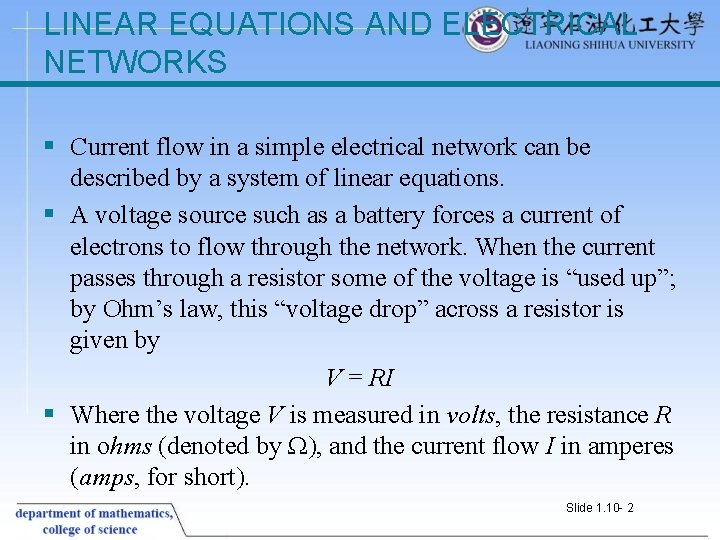LINEAR EQUATIONS AND ELECTRICAL NETWORKS § Current flow in a simple electrical network can be described by a system of linear equations. § A voltage source such as a battery forces a current of electrons to flow through the network. When the current passes through a resistor some of the voltage is “used up”; by Ohm’s law, this “voltage drop” across a resistor is given by V = RI § Where the voltage V is measured in volts, the resistance R in ohms (denoted by Ω), and the current flow I in amperes (amps, for short). Slide 1. 10 - 2LINEAR EQUATIONS AND ELECTRICAL NETWORKS § Kirchhoff’s Voltage Law: The algebraic sum of RI voltage drops in one direction around a loop equals the algebraic sum of the voltage sources in the same direction around the loop. § Example 2 Determine the loop currents in the network in Fig. 1 § Solution For loop 1, the current I 1 flows through three resistors, and the sum of the RI voltage drops is 4 I 1 + 3 I 1 = (4 + 3)I 1= 11 I 1 Slide 1. 10 - 3LINEAR EQUATIONS AND ELECTRICAL NETWORKS Slide 1. 10 - 4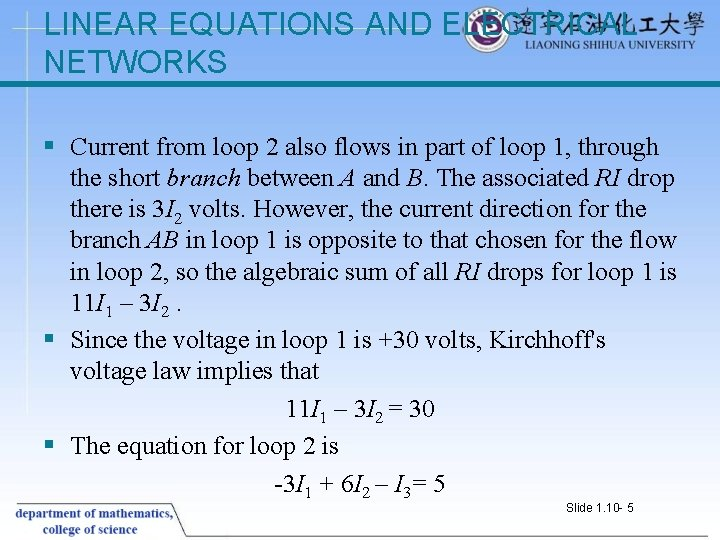LINEAR EQUATIONS AND ELECTRICAL NETWORKS § Current from loop 2 also flows in part of loop 1, through the short branch between A and B. The associated RI drop there is 3 I 2 volts. However, the current direction for the branch AB in loop 1 is opposite to that chosen for the flow in loop 2, so the algebraic sum of all RI drops for loop 1 is 11 I 1 – 3 I 2. § Since the voltage in loop 1 is +30 volts, Kirchhoff's voltage law implies that 11 I 1 – 3 I 2 = 30 § The equation for loop 2 is -3 I 1 + 6 I 2 – I 3= 5 Slide 1. 10 - 5LINEAR EQUATIONS AND ELECTRICAL NETWORKS § The term -3 I 1 comes from the flow of the loop-1 current through the branch AB (with a negative voltage drop because the current flow there is opposite to the flow in loop 2). § The term 6 I 2 is the sum of all resistances in loop 2, multiplied by the loop current. § The term –I 3 = -1·I 3 comes from the loop-3 current flowing through the 1 -ohm resistor in branch DC, in the direction opposite to the flow in loop 2. The loop-3 equation is -I 2 + 3 I 3= -25 Slide 1. 10 - 6LINEAR EQUATIONS AND ELECTRICAL NETWORKS § Slide 1. 10 - 7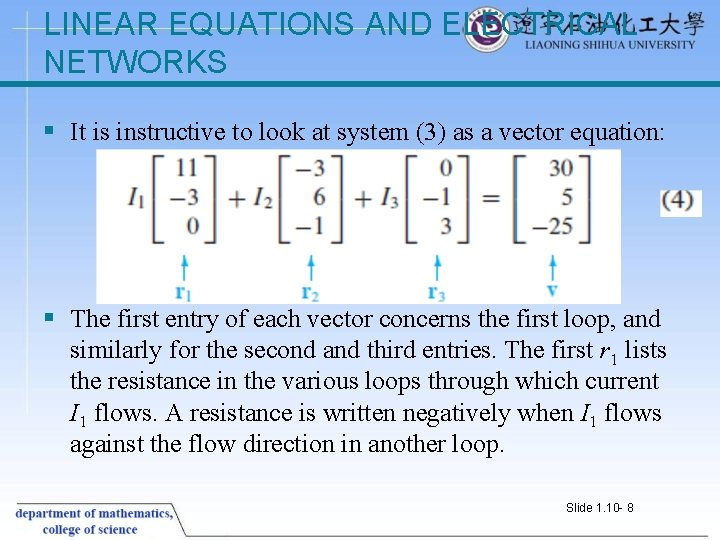LINEAR EQUATIONS AND ELECTRICAL NETWORKS § It is instructive to look at system (3) as a vector equation: § The first entry of each vector concerns the first loop, and similarly for the second and third entries. The first r 1 lists the resistance in the various loops through which current I 1 flows. A resistance is written negatively when I 1 flows against the flow direction in another loop. Slide 1. 10 - 8LINEAR EQUATIONS AND ELECTRICAL NETWORKS § Examine Fig. 1 and see how to compute the entries in r 1; then do the same for r 2 and r 3. The matrix form of equation (4), Provides a matrix version of Ohm’s law. If all loop currents are chosen in the same direction (say, counterclockwise), then all entries off the main diagonal of R will be negative. Slide 1. 10 - 9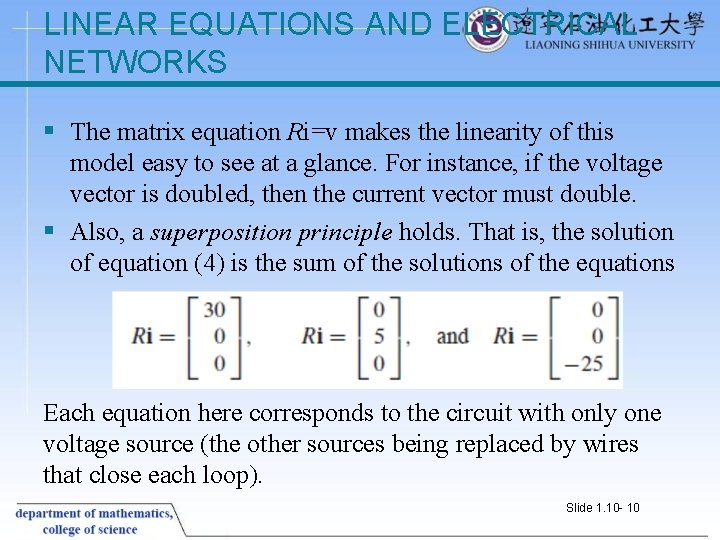LINEAR EQUATIONS AND ELECTRICAL NETWORKS § The matrix equation Ri=v makes the linearity of this model easy to see at a glance. For instance, if the voltage vector is doubled, then the current vector must double. § Also, a superposition principle holds. That is, the solution of equation (4) is the sum of the solutions of the equations Each equation here corresponds to the circuit with only one voltage source (the other sources being replaced by wires that close each loop). Slide 1. 10 - 10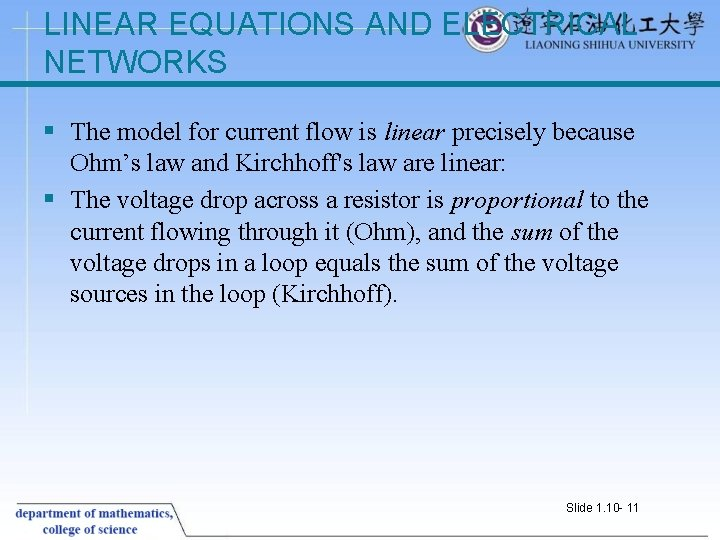LINEAR EQUATIONS AND ELECTRICAL NETWORKS § The model for current flow is linear precisely because Ohm’s law and Kirchhoff's law are linear: § The voltage drop across a resistor is proportional to the current flowing through it (Ohm), and the sum of the voltage drops in a loop equals the sum of the voltage sources in the loop (Kirchhoff). Slide 1. 10 - 11LINEAR EQUATIONS AND ELECTRICAL NETWORKS § Slide 1. 10 - 12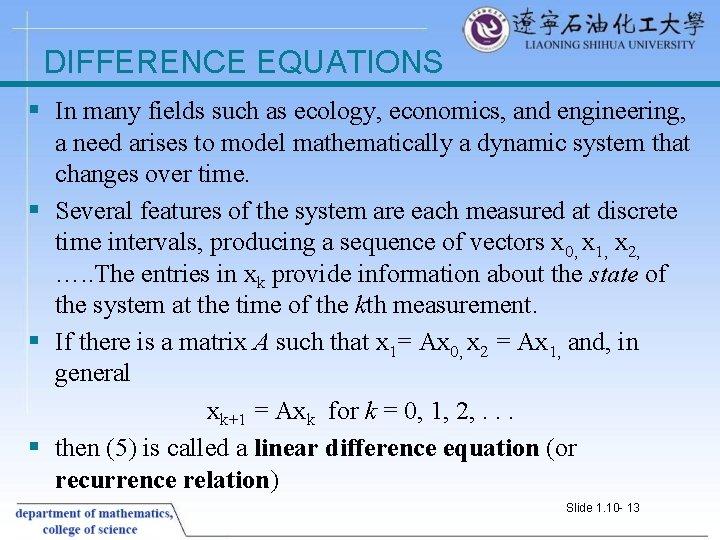DIFFERENCE EQUATIONS § In many fields such as ecology, economics, and engineering, a need arises to model mathematically a dynamic system that changes over time. § Several features of the system are each measured at discrete time intervals, producing a sequence of vectors x 0, x 1, x 2, …. . The entries in xk provide information about the state of the system at the time of the kth measurement. § If there is a matrix A such that x 1= Ax 0, x 2 = Ax 1, and, in general xk+1 = Axk for k = 0, 1, 2, . . . § then (5) is called a linear difference equation (or recurrence relation) Slide 1. 10 - 13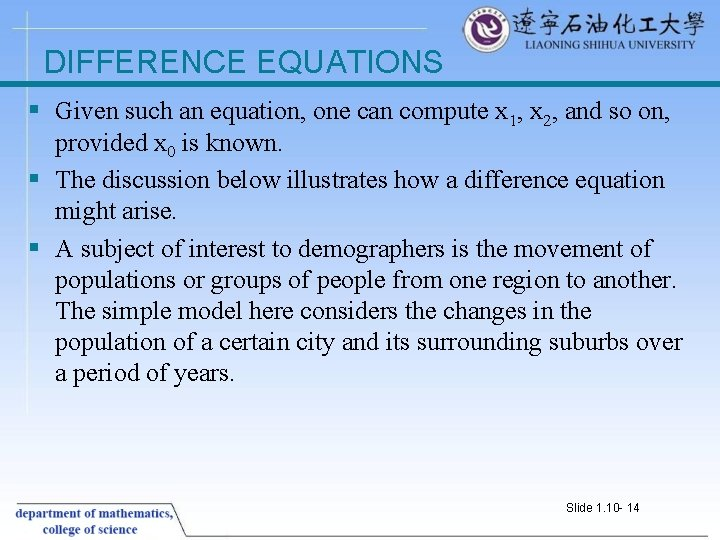DIFFERENCE EQUATIONS § Given such an equation, one can compute x 1, x 2, and so on, provided x 0 is known. § The discussion below illustrates how a difference equation might arise. § A subject of interest to demographers is the movement of populations or groups of people from one region to another. The simple model here considers the changes in the population of a certain city and its surrounding suburbs over a period of years. Slide 1. 10 - 14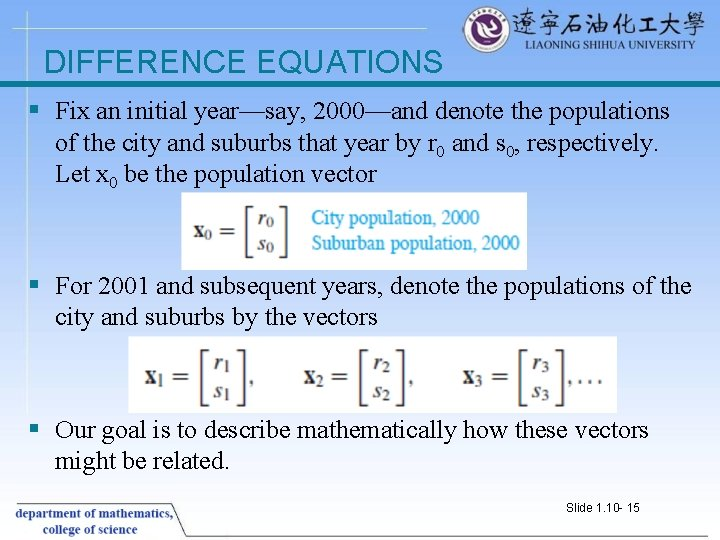DIFFERENCE EQUATIONS § Fix an initial year—say, 2000—and denote the populations of the city and suburbs that year by r 0 and s 0, respectively. Let x 0 be the population vector § For 2001 and subsequent years, denote the populations of the city and suburbs by the vectors § Our goal is to describe mathematically how these vectors might be related. Slide 1. 10 - 15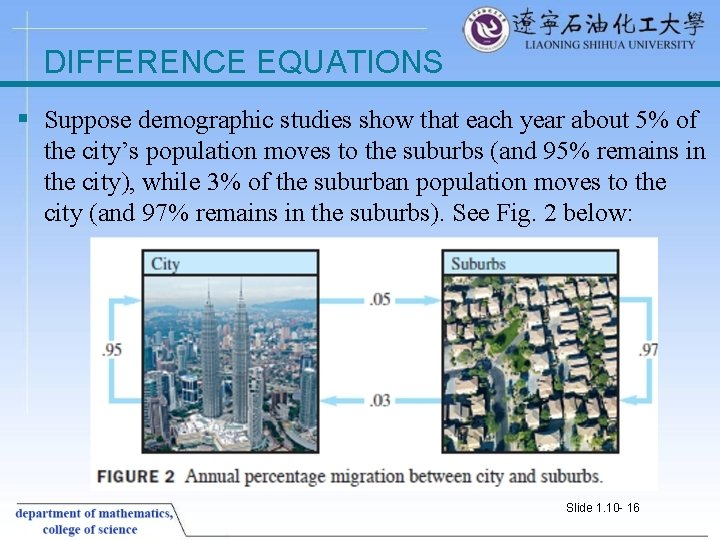DIFFERENCE EQUATIONS § Suppose demographic studies show that each year about 5% of the city’s population moves to the suburbs (and 95% remains in the city), while 3% of the suburban population moves to the city (and 97% remains in the suburbs). See Fig. 2 below: Slide 1. 10 - 16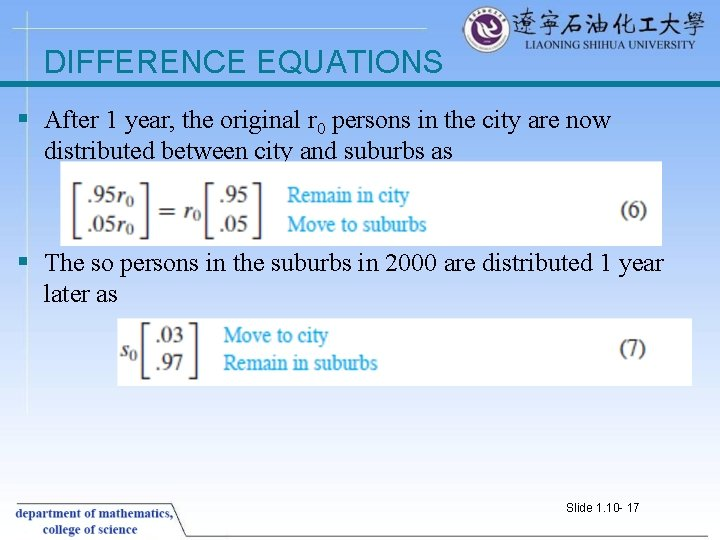DIFFERENCE EQUATIONS § After 1 year, the original r 0 persons in the city are now distributed between city and suburbs as § The so persons in the suburbs in 2000 are distributed 1 year later as Slide 1. 10 - 17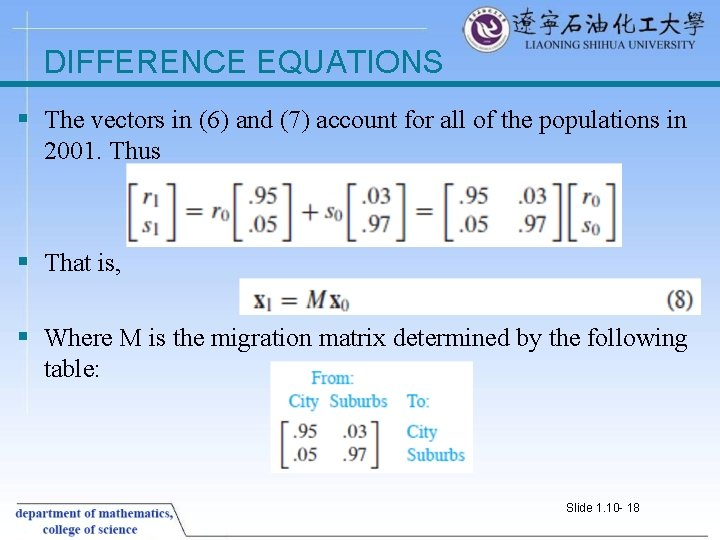DIFFERENCE EQUATIONS § The vectors in (6) and (7) account for all of the populations in 2001. Thus § That is, § Where M is the migration matrix determined by the following table: Slide 1. 10 - 18DIFFERENCE EQUATIONS § Equation (8) describes how the population changes from 2000 to 2001. If the migration percentages remain constant, then the change from 2001 to 2002 is given by § And similarly for 2002 to 2003 and subsequent years. In general, § The sequence of vectors {x 0, x 1, x 2, …} describes the population of the city/suburban region over a period of years. Slide 1. 10 - 19